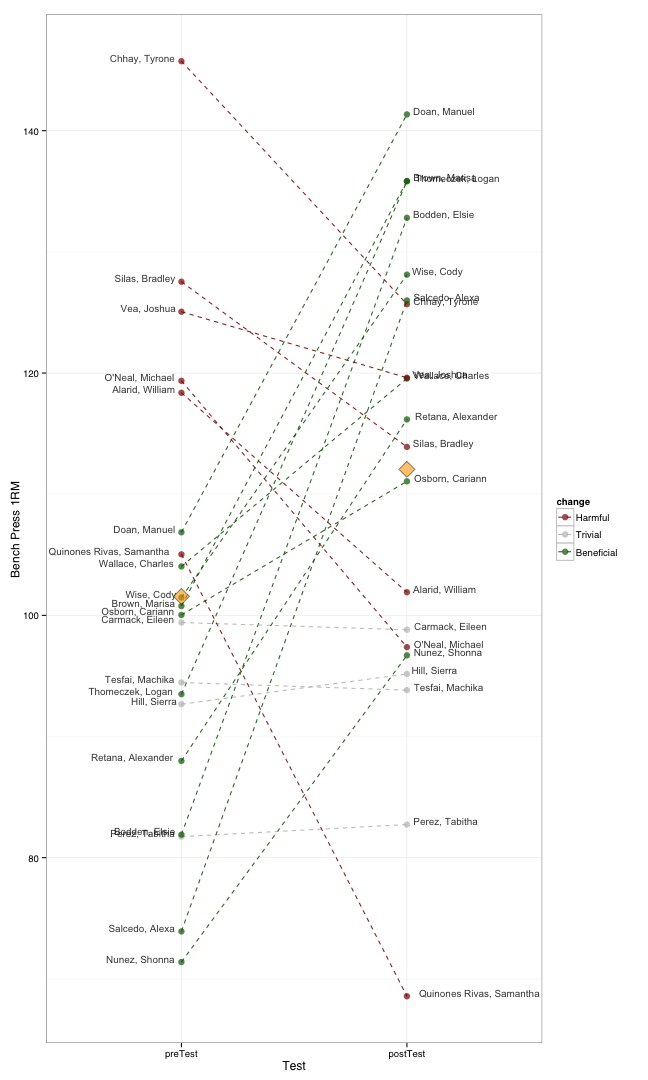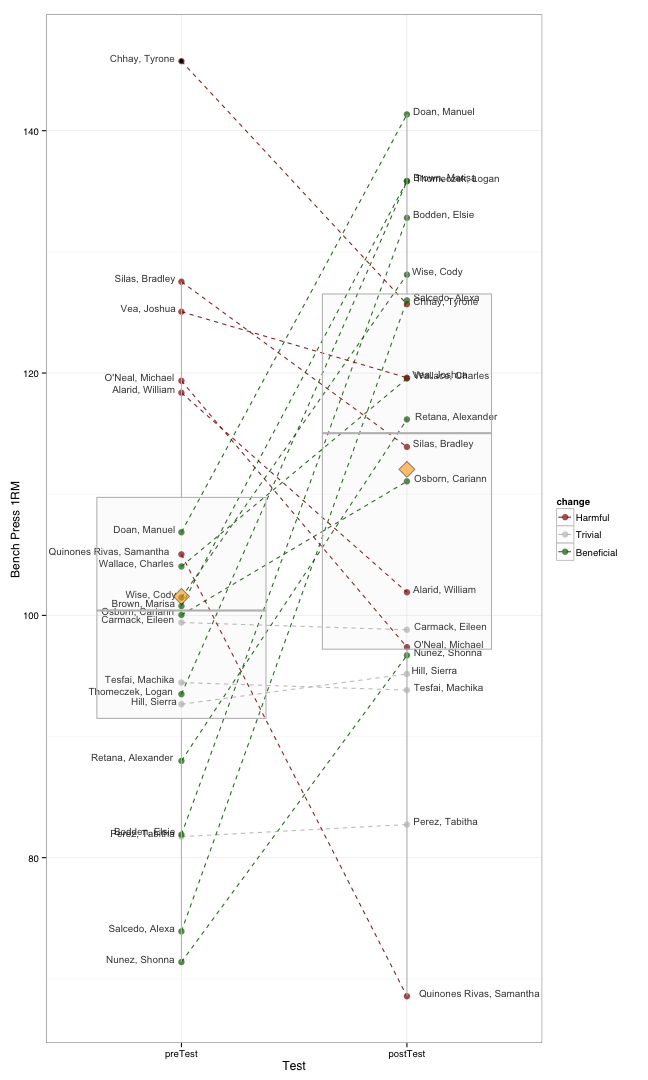## Thursday, December 26, 2013

### How to visualize test change scores for coaches. Part 2

How to visualize test change scores for coaches. Part 2

# How to visualize test change scores for coaches. Part 2

To continue the previous How to visualize test change scores for coaches blogpost, here is another way to visualize individual change scores without falling for group averages. This way we can easily see individual ranks in both pre- and post- test, along with change score (which is also color coded). Quite easy to identify the outliers.

Orange rectanges represent group averages.

Enjoy!

``````library(reshape2)
library(ggplot2)
library(randomNames)

set.seed(112)

numberOfAthletes <- 20

athletes <- randomNames(numberOfAthletes)

test1 <- rnorm(mean = 100, sd = 20, n = numberOfAthletes)
test2 <- rnorm(mean = 110, sd = 20, n = numberOfAthletes)
diff <- test2 - test1

SWC <- sd(test1) * 0.2

change <- rep("Trivial", numberOfAthletes)

change[diff > SWC] <- "Beneficial"
change[diff < -SWC] <- "Harmful"

change <- factor(change, c("Harmful", "Trivial", "Beneficial"), ordered = T)

testingData <- data.frame(athlete = athletes, preTest = test1, postTest = test2,
diff = diff, change = change)

testingData <- melt(testingData, id.vars = c("athlete", "diff", "change"))

g <- ggplot(testingData, aes(x = variable, y = value)) + geom_line(aes(group = athlete,
color = change), linetype = "dashed", alpha = 0.9)

g <- g + geom_point(aes(color = change), size = 3, alpha = 0.7) + theme_bw(base_size = 12,
base_family = "Helvetica") + scale_colour_manual(values = c("dark red",
"grey", "dark green"))

g <- g + geom_text(data = subset(testingData, variable == "preTest"), aes(x = variable,
label = athlete, y = value), alpha = 0.8, size = 3.5, hjust = 1.1, vjust = 0)

g <- g + geom_text(data = subset(testingData, variable == "postTest"), aes(x = variable,
label = athlete, y = value), alpha = 0.8, size = 3.5, hjust = -0.1, vjust = 0)

g <- g + labs(x = "Test", y = "Bench Press 1RM")

g <- g + stat_summary(fun.y = mean, geom = "point", fill = "orange", shape = 23,
size = 6, alpha = 0.6)
g
``````And we can even put boxplot on top of it

``````g <- g + geom_boxplot(color = "grey", fill = "grey", alpha = 0.05)
g
``````